# WAEC General Mathematics Theory Paper 2, May/June. 2015 Past Question and Answers

See the WAEC General Mathematics Theory Paper 2, May/June. 2015 Past Question and Answers here. See the questions and the general answers from the marking scheme here.

Question 1

(a)  Without using Mathematical tables or calculator, simplify
3¸ (5– 2) + 5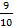.

(b)  A number is selected at random from each of the sets {2, 3, 4} and {1, 3, 5}.
Find the probability that the sum of the two numbers is greater than 3 and less
than 7.

### Observation

The Chief Examiner reported that this question was attempted by majority of the candidates and their performance was better in part (a) than in part (b).
In part (a), candidates’ performance was commended. Majority of them were reported to apply the rule of BODMAS correctly. Candidates were expected to simplify the bracket first to obtain 5– 2=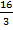–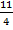=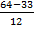=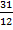. Therefore, 3÷ (5– 2) + 5became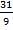÷+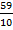=×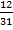+=+= 7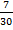.
In part (b), it was reported that candidates’ performance was not as good as it was in part (a). Majority of them were not able to obtain the sample space of the outcomes which was tabulated as follows

 2 3 4 1 1,2 1,3 1,4 3 3,2 3,3 3,4 5 5,2 5,3 5,4

From the table, the total number of possible outcomes = 9. The favourable outcomes were {(1, 3), (1, 4), (3, 2) and (3, 3)}. Therefore, the number of favourable outcomes = 4. Hence, the required probability =.

Question 2

(a)  Solve the inequality: 4 + ¾ (x + 2)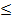3/8x + 1
(b)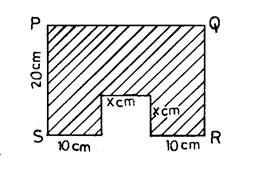The diagram shows a rectangle PQRS from which a square of side x cm has been cut.  If the area of the shaded portion is 484 cm2, find the values of x.

### Observation

The Chief Examiner reported that this question was also attempted by majority of the candidates.

In part (a), majority of the candidates were reported to have correctly multiplied through the inequality by the LCM of denominators, which was 8, to obtain 32 + 6(x + 2) ≤ 3x + 8. Simplifying this inequality gave the answer x ≤ -12.

In part (b), a good number of the candidates were reported not to interpret the question correctly and as a result could not obtain the resulting equation. Candidates were expected to show that the area of the shaded portion = area of rectangle – area of the square. Here, length of rectangle = (20 + x) cm and breadth = 20 cm. Therefore, 484 cm2 = (20 + x)(20) – x2. Expanding the bracket and simplifying gave the equation x2 – 20x + 84 = 0. Solving this equation gave the values of x as 6 cm and 14 cm.

Question 3

(a)  The ratio of the interior angle to the exterior angle of a regular polygon is 5 : 2.
Find the number of sides of the polygon.
(b)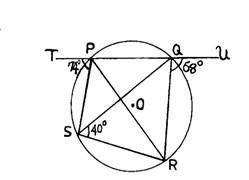The diagram shows a circle PQRS with centre O, ÐUQR = 68o
ÐTPS = 74o and ÐQSR = 40o.  Calculate the value of ÐPRS.

### Observation

This question was also reported to be very popular among the candidates but they performed poorly in it. According to the report, their responses indicated a poor knowledge of geometry and teachers were encouraged to emphasize this area of the syllabus.

In part (a), candidates were expected to recall the formula for the sum of the interior angles and exterior angles of a polygon respectively. However, majority of them were reported not to show that each interior angle of a regular polygon was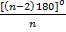while each exterior angle was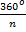.Since the ratio was 5 : 2, it implied that=i.e.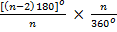=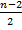=. Simplifying gave n = 7.

In part (b), majority of the candidates were reported not to apply the circle theorems correctly.They were expected to show that from the diagram, ∠RSQ = ∠RPQ (angles in the same segment). This implied that ∠PRQ = 68o – 40o = 28o (exterior angle of triangle PQR = sum of the two opposite interior angle). ∠TPS = ∠SRQ = 74o (TPS is the exterior angle of cyclic quadrilateral PQRS). Therefore, the required angle PRS = 74o – 28o = 46o

We have complied all the WAEC Past Questions and Amswers on mathematics. Click to buy your copy now.